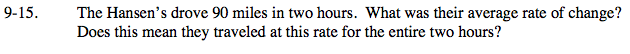### Home > AC > Chapter 16 > Lesson 16.9.1.1 > Problem9-15

9-15.

The Hansen’s drove 90 miles in two hours. What was their average rate of change? Does this mean they traveled at this rate for the entire two hours? Homework Help ✎$\text{average rate }= \frac{\text{total distance}}{\text{total time}}$